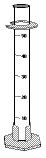# Volume of Irregular Object Lab

Volume of an Irregular-shaped ObjectIntroduction

The word mass is used to tell how much matter there is in something. Matter is anything you can touch physically. An electronic scale or triple beam balance can be used to tell the mass of an object. Volume is a measure of how much space an object occupies. When measuring the volume of a liquid, a graduated cylinder can be used.  Measurement is the collection of quantitative data (numbers). Measurements are not only numbers. They must always contain a unit of measurement. In the Metric System, the gram (g) is the basic unit of measurement for mass. The basic unit of measurement for the volume of liquids is the milliliter (ml). The density of an object can be determined by dividing the mass by the volume (D = M/V). From a density calculation, we may tell whether a substance will float or sink in another liquid. A less dense substance will float on one that is denser. An example is oil floating on water. Oil is less dense than the water.

For solids that have an irregular shape, the displacement method must be used to determine their volume. When using the displacement method, you must first measure the starting volume of the liquid. Then add the object and record the change in volume. This gives the volume of the irregularly-shaped object.

Hypothesis

The density of objects can be determined by a method known as water displacement.

Materials

Triple beam or electronic balance
3 irregularly shaped objects
calculator
pencil

Methods

1. Obtain 3 irregularly shaped objects that will sink in water.
2. Estimate the mass & volume of each object. Record this on table 1.
3. Use a triple beam balance or electronic scale to determine the mass in grams of each object. Record this on table 1.
4. Fill a graduated cylinder halfway with tap water.
5. Measure & record the volume of the water in the graduated cylinder. READ THE MENISCUS!
6. Place one of the objects into the graduated cylinder, and measure and record the new volume.
7. Subtract the initial volume from the final volume. The difference is the volume of the object.
8. Record the difference in data table 1.
9. Repeat steps 4 – 8 for the other three objects.
10. To determine the density of each object, divide the actual mass of each object by its volume (determined by water displacement).
11. Record the densities in data table 1.

Results & Data

Data Table 1

 Object Estimated Mass (g) Estimated Volume (ml) Actual Mass (g) Volume of H2O in graduated cylinder (ml) Volume of H2O and object in graduated cylinder (ml) Object’s Volume (Subtract Column 5 from Column 6) (ml) Density D=m/V (Divide Column 4 by Column 7) (g/ml)

Questions

1. How did you determine the object’s:

a. mass?

b. Volume?

c. density?

1. Objects will sink if they are denser than water. Explain why ships made of steel float instead of sinking since steel is denser than water.

Word Document